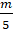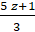Daily Practice Problems
Class 7 Maths
Simple EquationsQuestion 1:

Convert the following equations in statement form:

(i) x – 5 = 9

(ii) 5p = 20

(iii) 3n + 7 = 1

(iv)– 2 = 6

Question 2:

Find a number, such that one-fourth of the number is 3 more than 7.

Question 3:

Solve the following equations:

3(y – 2) = 2(y – 1) – 3

Question 4:

Manu’s father’s age is 4 years more than three times Manu’s age. Find Manu’s age, if his father is 37 years old.

Question 5:

Solve:

(a) 4(m + 3) = 18

(b) – 2(x + 3) = 8

Question 6:

When you multiply a number by 6 and subtract 5 from the product, you get 7. Can you tell what the number is?

Question 7:

In a mathematics quiz, 30 prizes consisting 1st and 2nd are only to be given. 1st and 2nd prizes are worth Rs 2000 and Rs 1000 respectively. If the total prize money is Rs 52,000 then find the number of 1st prizes and 2nd prizes.

Question 8:

The length of a rectangle is twice its breadth. If its perimeter is 60 cm, find the length and the breadth of the rectangle.

Question 9:

The sum of three consecutive multiples of 2 is 18. Find the numbers.

Question 10:

Each of the 2 equal sides of an isosceles triangle is twice as large as the third side. If the perimeter of the triangle is 30 cm, find the length of each side of the triangle.

Question 11:

Solve:

(a) 3n + 7 = 25

(b) 2p – 1 = 23

Question 12:

Check whether the value given in brackets is solution to the given equation or not.

a) x – 7 = 17 (x = 10)

b) y + 8 = 10 (y =2)

Question 13:

Raju’s father’s age is 5 years more than three times Raju’s age. Find Raju’s age, if his father is 44 years old.

Question 14:

Solve the following equation:= 7

Question 15:

Write equations for the following statements:

a) 3 subtracted from 2 times of a number p is 9.

b) Ten times a is 30.

Question 16:

If one-third of a number exceeds its one-fourth by 1, find the number.

Question 17:

Seven times a number is 12 less than thirteen times the same number. Find the number.

Question 18:

In a test, Abha gets twice the marks as that of Palak. Two times Abha’s marks and three times Palak’s marks make 280. Find Palak and Abha’s marks.

Question 19:

If the interest received by Karim is Rs 30 more than that of Ramesh. If the total interest received by them is Rs 70, Find the interest received by Ramesh.

Question 20:

After 20 years, Manoj will be 5 times as old as he is now. Find his present age.

**********